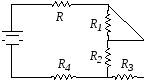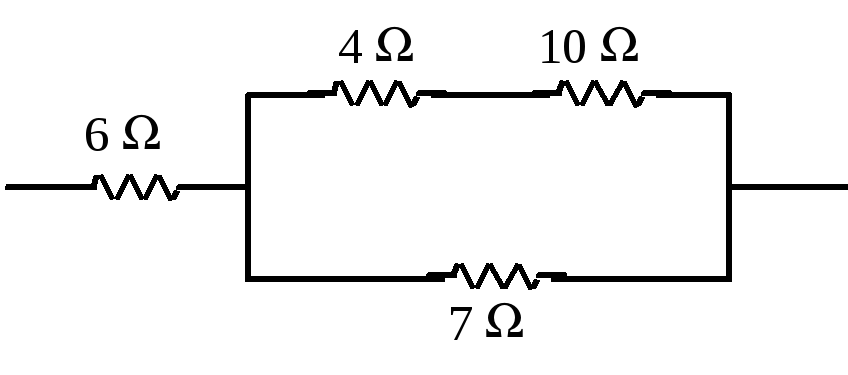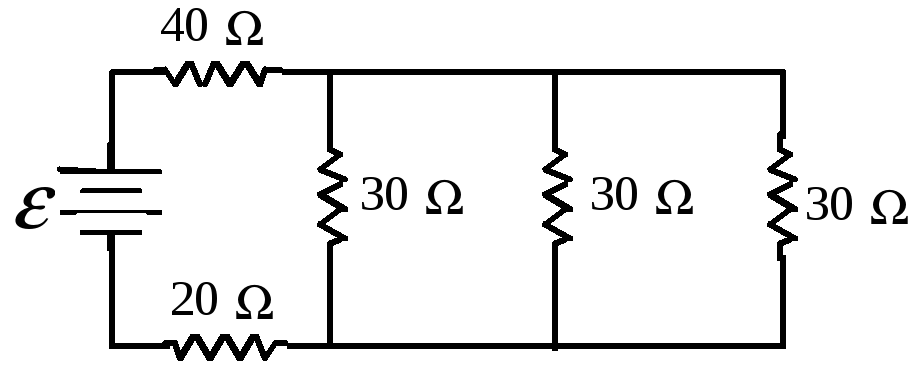# Phy 2054 Discussion – Fall ‘08 Practice Exam Problems (Chapter 18)

 Last updated: September 30, 2008 PHY 2054 Discussion – Fall ‘08 Practice Exam Problems (Chapter 18) When a 24.0-Ω resistor is connected across a 12.0-V battery, a current of 482 mA flows. What is the resulting terminal voltage of the battery? (Emf) a. 0.4 V b. 5.8 V c. 11.6 V d. 12.0 V If R123, and if these resistors are connected in series in a circuit, which one dissipates the greatest power? (Resistors in Series) a. R1 b. R2 c. R3 d. All are equal in power dissipation Which two resistors are in parallel with each other? (Resistors in Parallel) a. R and R4 b. R2 and R3 c. R2 and R4 d. R and R1Resistors of values 6.0 Ω, 4.0 Ω, 10.0 Ω and 7.0 Ω are combined as shown. What is the equivalent resistance for this combination? (Equivalent resistance) a. 2.3 Ω b 3.0 Ω c. 10.7 Ω d. 27 ΩIf ε = 20 V, at what rate is thermal energy being generated in the 20-Ω resistor? (Combinations of resistors) a. 6.5 W b. 1.6 W c. 15 W d. 26 WWhat is the current through the 2-Ω resistor? (Complex DC circut) a. 1.0 A b. 0.50 A c. 1.5 A d. 2.0 A Share with your friends: